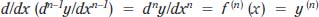NextPrevious

# Are there higher derivatives of a function?

Yes, there are higher derivatives of a function, often referred to as higher order derivatives. The “initial” derivative is often written as f' (x), but the “ ' ” is assumed in most cases. The next derivative is the second derivative (second order derivative), most often written as f'' (x); the next is the third derivative, or third order derivative, most often written as f''' (x); fourth derivative, or fourth order derivative, most often written as f(4)(x); and so on. The notation for the higher derivatives, or the nth derivative, is as follows:This equation is also seen written as Dn (y) = dny/dxn.

Close

This is a web preview of the "The Handy Math Answer Book" app. Many features only work on your mobile device. If you like what you see, we hope you will consider buying. Get the App